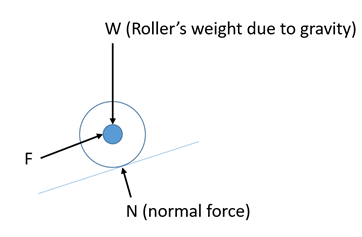## Free Body Diagram

Draw the free body diagram of the below roller being held in place on a frictionless inclined plane.Hint
A force is a vector quantity where magnitude, point of application, and direction are all known.
Hint 2
There are three forces on the roller: force being applied by the person, the roller's weight due to gravity, and the normal force.
A force is a vector quantity where magnitude, point of application, and direction are all known. There are three forces on the roller: force being applied by the person, the roller's weight due to gravity, and the normal force. Gravity always points directly downward and the normal force is always perpendicular to the contact surface. The applied force is where the person is holding the roller in place.